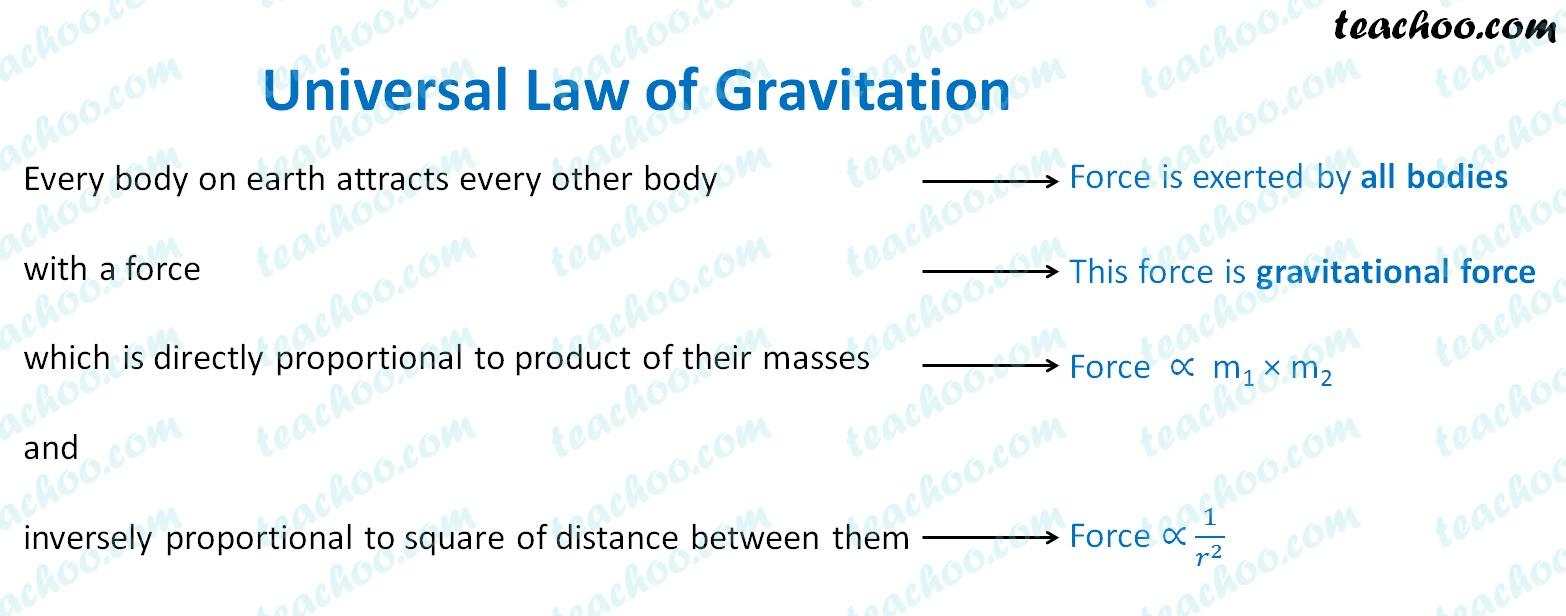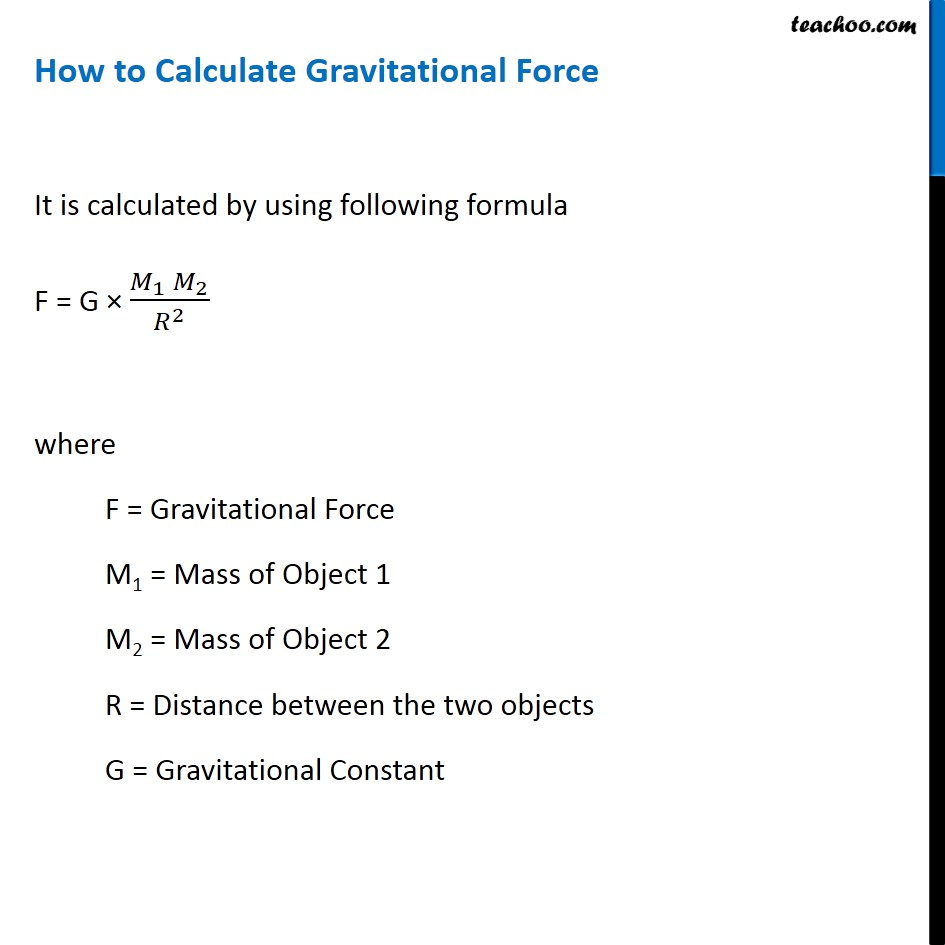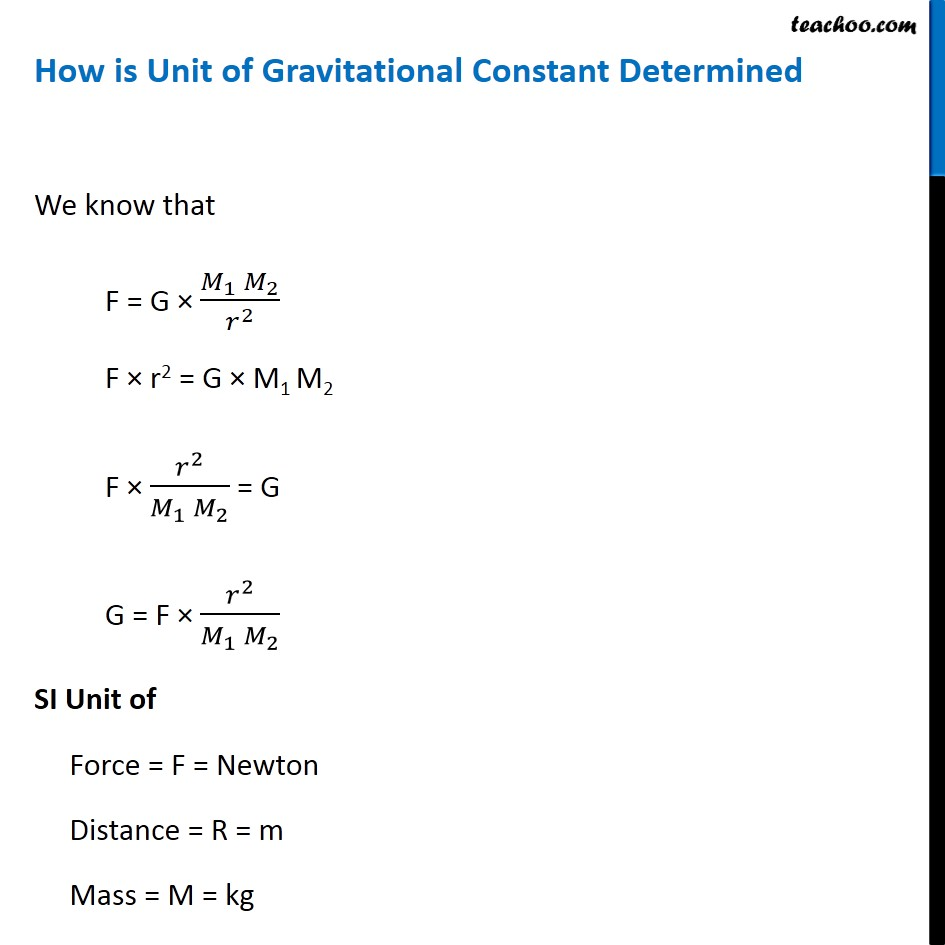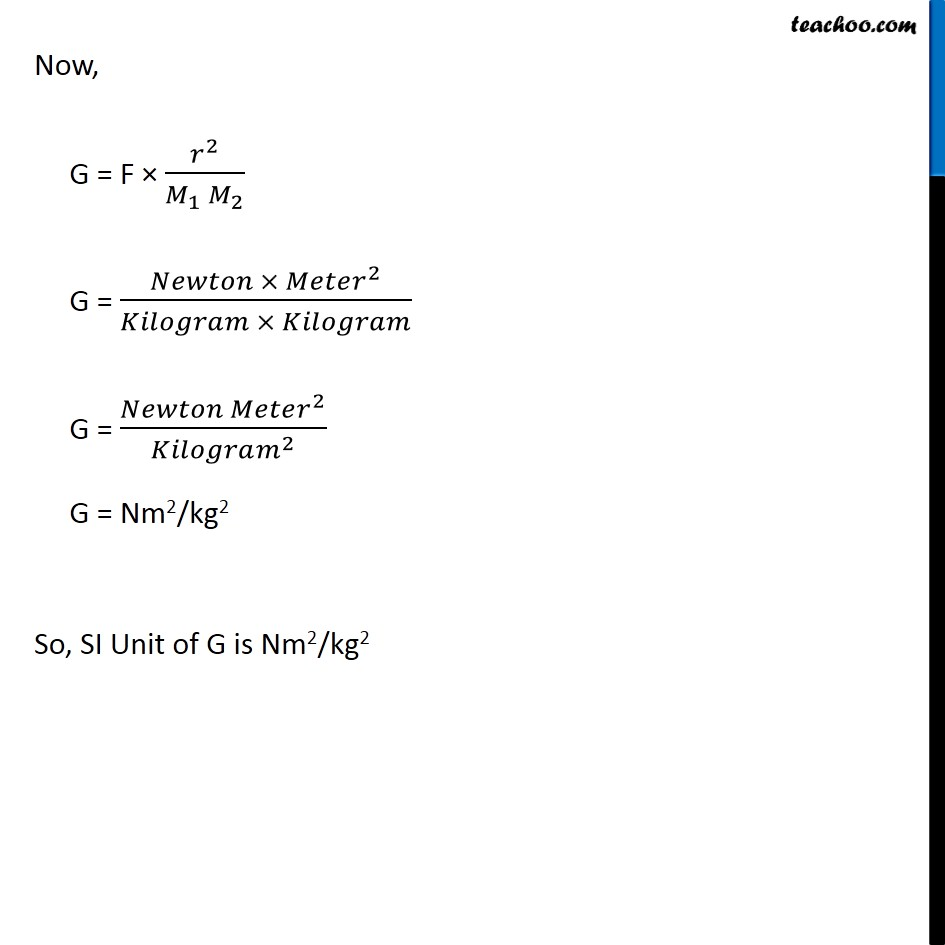Concepts

Class 9
Chapter 10 Class 9 - Gravitation

## According to Newton's Law of Gravitation

Every body on earth attracts every other body

with a force

which is directly proportional to product of their masses and  inversely proportional to square of distance between them## Important Points

### Gravitational Force is directly proportional to product of masses of 2 objects

Meaning

If mass of body is less, there is less gravitational force

2 small stones lying on ground exert very small gravitational force on each other

If mass of body is more, there is more gravitational force

Stone and earth exert high gravitational force because product of masses is high

How to Show it by  Formula

Let Gravitational Force be F

Mass of Body 1 = m 1

Mass of body 2 = m 2

Hence, this can be depicted as

Force ∝ m 1  × m 2

Analysis

If we double the mass of one object,gravitational force becomes double

If we double mass of both objects, gravitational force becomes 4 times

## Gravitational force is inversely proportional to square of distance between them

Let distance between two objects be r

Force ∝ 1/r 2

Hence,more the distance between 2 objects, less the gravitational force

There is very less gravitational force of stars on earth because distance between earth and star is very large

Less distance between 2 objects more the gravitational force

There is very high gravitational force of sun on earth because distance between earth and sun is comparatively less

How to Show by Formula

Let distance between two objects be r

Force ∝ 1/r 2

Analysis

If we double the distance between 2 objects, Gravitational Force becomes 1/4 times

If we triple the distance between 2 objects, Gravitational Force becomes 1/9 times## What is Gravitational Constant?

It is the constant value which is taken while calculating gravitational force between 2 objects in universe

It is the gravitational force which exists between 2 bodies of 1 kg each are kept at distance of 1 meter from each other

This force of gravitational force has been calculated to be very small 6.67 × 10 -11 N m kg -2

G = 6.67 × 10 -11 N m kg -2## Questions

Q 1 Page 134 - State the universal law of gravitation.

Q 2 Page 134 - Write the formula to find the magnitude of the gravitational force between the earth and an object on the surface of the earth.

Example 10.1 - The mass of the earth is 6 × 1024 kg & that of the moon is 7.4 × 1022 kg. If the distance between the earth and the moon is 3.84 × 105 km, calculate the force exerted by the earth on the moon. (Take G = 6.7 × 10 –11 N m 2 kg −2 )

NCERT Question 1 - How does the force of gravitation between two objects change when the distance between them is reduced to half ?

NCERT Question 2 - Gravitational force acts on all objects in proportion to their masses. Why then, a heavy object does not fall faster than a light object?

NCERT Question 3 - What is the magnitude of the gravitational force between the earth and a 1 kg object on its surface? (Mass of the earth is 6 × 1024 kg and radius of the earth is 6.4 × 106 m.)

NCERT Question 4 - The earth and the moon are attracted to each other by gravitational force. Does the earth attract the moon with a force that is greater or smaller or the same as the force with which the moon attracts the earth? Why?

NCERT Question 5 - If the moon attracts the earth, why does the earth not move towards the moon?

NCERT Question 6 - What happens to the force between two objects, if (i) the mass of one object is doubled? (ii) the distance between the objects is doubled and tripled? (iii) the masses of both objects are doubled?

NCERT Question 16 - Calculate the force of gravitation between the earth & the Sun, given that the mass of the earth = 6 × 10 24 kg and of the Sun = 2 × 10 30 kg. The average distance between the two is 1.5 × 10 11 m.

Learn in your speed, with individual attention - Teachoo Maths 1-on-1 Class

### Transcript

Universal Law of Gravitation Every body on earth attracts every other body with a force which is directly proportional to product of their masses and inversely proportional to square of distance between them Force is exerted by all bodies This force is gravitational force Force ∝ 1/𝑟^2 How to Calculate Gravitational Force It is calculated by using following formula F = G × (𝑀_1 𝑀_2)/𝑅^2 where F = Gravitational Force M1 = Mass of Object 1 M2 = Mass of Object 2 R = Distance between the two objects G = Gravitational Constant How is Unit of Gravitational Constant Determined We know that F = G × (𝑀_1 𝑀_2)/𝑟^2 F × r2 = G × M1 M2 F × 𝑟^2/(𝑀_1 𝑀_2 ) = G G = F × 𝑟^2/(𝑀_1 𝑀_2 ) SI Unit of Force = F = Newton Distance = R = m Mass = M = kg Now, G = F × 𝑟^2/(𝑀_1 𝑀_2 ) G = (𝑁𝑒𝑤𝑡𝑜𝑛 × 𝑀𝑒𝑡𝑒𝑟^2)/(𝐾𝑖𝑙𝑜𝑔𝑟𝑎𝑚 × 𝐾𝑖𝑙𝑜𝑔𝑟𝑎𝑚) G = (𝑁𝑒𝑤𝑡𝑜𝑛 𝑀𝑒𝑡𝑒𝑟^2)/(𝐾𝑖𝑙𝑜𝑔𝑟𝑎𝑚^2 ) G = Nm2/kg2 So, SI Unit of G is Nm2/kg2